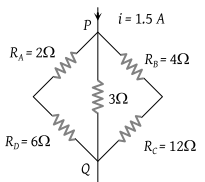Potential difference between the points P and Q in the electric circuit shown is(1) 4.5 V

(2) 1.2 V

(3) 2.4 V

(4) 2.88 V

(4) Equivalent resistance between P and Q

$\frac{1}{{R}_{PQ}}=\frac{1}{\left(6+2\right)}+\frac{1}{3}+\frac{1}{\left(4+12\right)}$${R}_{PQ}=\frac{48}{25}\Omega$

Current between P and Q; i = 1.5A

So, potential difference between P and Q

${V}_{PQ}=1.5×\frac{48}{25}=2.88V$

Difficulty Level:

• 23%
• 30%
• 10%
• 38%